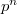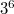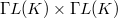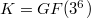﻿

### On a conjecture about the autotopism group of the Figueroa's presemifields of order$p^n$

#### Abstract

In  was proved that the autotopism group of the Cordero-Figueroa semifield of order$3^6$ is isomorphic to a subgroup of$\Gamma L(K) \times \Gamma L(K)$, where$K = GF(3^6)$. Also a conjecture was proposed for the general case, the autotopism group of a Figueroa's presemifield of order$p^n$. In this article, under a normality condition, we prove this conjecture.

DOI Code: 10.1285/i15900932v38n2p11

Keywords: finite presemifield; finite semifield; autotopism; autotopism group; Cordero-Figueroa semifield; Figueroa's presemifields

Full Text: PDF